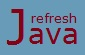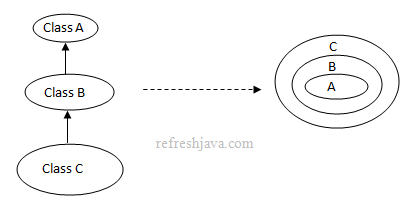# Polymorphism in Java - Definition, Example & Advantages

Polymorphism is another fundamental principal of object-oriented programming. Sometimes beginners find it little difficult to understand what exactly the polymorphism is, so we will try to see this with some real world examples and programs to understand this easily. This tutorial covers different aspect of polymorphism like what is polymorphism, real world example, advantages of polymorphism etc.

## What is Polymorphism

The word polymorphism is made from two words, `poly` which means `many` and `morphism` which means `forms or types`, so the word polymorphism means many forms. Polymorphims in java is a mechanism in which an object or it's behavior can have many different forms, we call such objects as polymorphic object.

Programmatically, in java the object of a child class can be assigned in any of its parent classes in the inheritance hierarchy which means the object of a subclass can take the form of any of it's parent classes in it's inheritance hierarchy, so we can say all the parent classes are basically different forms of child class, which is what the polymorphism is.

For example let's consider a scenario in which we have class A, B and C where class C extends class B which extends class A. In this case the object of class C can be assigned into class C, class B or class A which means the object of class C can take form of it's own, class B or class A.```  C ob = `new` C();
B b = c;   `or` B b = `new` C(); `// C class object will act as B class object`
A a = c;   `or` A a = `new` C(); `// C class object will act as A class object`
```

As you can see object of class C can be assigned into any of it's parent class which means class C object can take forms of class B and class A as well along with it's own. This means object of C class is polymorphic in nature. The same happens with class B object as well which can take the form of it's own and class A.

## Real world example of Polymorphism

To take a real world example, we can consider ourself. As a person we have many different forms like student, teacher, player, father/mother etc. The same person can be a teacher as well as a player, so we can say person object is polymorphic in nature.

Another real world example is your mobile. Sometime your mobile behaves as a phone, sometime as a camera, sometime as a radio etc. Here the same mobile phone has different forms, so we can say the mobile object is polymorphic in nature.

## Java program of polymorphism

``` `class` Person {
String name;

`void` walk() {
System.out.println(`"My name is "`+ name+ `" and I can walk"`);
}
`void` talk() {
System.out.println(`"My name is "`+ name+ `" and I can talk"`);
}
}

`class` Teacher `extends` Person {
String subject;

`void` teach() {
System.out.println(`"I teaches "`+subject);
}
`public static void` main(String [] args) {
Teacher teacher = `new` Teacher(); `// teacher object having it's own form`
teacher.name = `"Rahul"`;
teacher.subject = `"Math"`;
teacher.walk();
teacher.talk();
teacher.teach();

Person person = teacher; `// teacher object takes the form of person object`
person.walk();
person.talk();
`//person.teach(); ``// Can't call teach method as it's not in Person class`
}
}
```

Output:

```My name is Rahul and I can walk
My name is Rahul and I can talk
I teaches Math
My name is Rahul and I can walk
My name is Rahul and I can talk
```

Here the object of teacher class is polymorphic as it can take two forms, one as itself and other as person. Remember if an object of subclass is behaving as parent class, then it can call the behaviors available in parent class only. In above example once the teacher is behaving as person, it can call only `walk` and `talk` behavior.

## Checking if an object is polymorphic.

Any object which have more than one `Is-A` relationship will be called as polymorphic object. For example in the scenario of class A, B, C given above, following observation will be true.

```  C is-a C
C is-a B
C is-a A
B is-a B
B is-a A
```

So the object of class C and class B will be polymorphic as they passes more than one is-a relationship. But what about if we have the object of class A, will that be polymorphic ?. The answer is yes, that will also be polymorphic. The reason is, every class in java internally extends `Object` class if it has not extended any other class, so in above case class A will internally extend `Object` class, this way the object of class A will also be polymorphic.

So in java every object(except the object of Object class) is polymorphic, no matter whether it's an object of user defined class or java's predefined class.

An is-a relation in java can be tested using `instanceof` operator. If an object passes more than one `instanceof` test, that object will be polymorphic.

```  C ob = `new` C();
if(ob `instanceof` C) `// true`
if(ob `instanceof` B) `// true`
if(ob `instanceof` A) `// true`
```